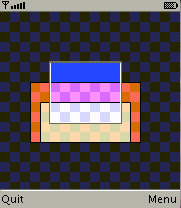-=+=- -=+=- -=+=- -=+=- -=+=- -=+=- -=+=- -=+=- -=+=- -=+=- -=+=- -=+=- -=+=- -=+=- -=+=- -=+=- -=+=- -=+=- -=+=- -=+=- -=+=- -=+=- -=+=- -=+=- -=+=- -=+=- -=+=- -=+=- -=+=- -=+=- (c) WidthPadding Industries 1987 0|267|0 -=+=- -=+=- -=+=- -=+=- -=+=- -=+=- -=+=- -=+=- -=+=- -=+=- -=+=- -=+=- -=+=- -=+=- -=+=- -=+=- -=+=- -=+=- -=+=- -=+=- -=+=- -=+=- -=+=- -=+=- -=+=- -=+=- -=+=- -=+=- -=+=- -=+=-
SoCoder -> Showcase Home -> Puzzle

PioCreated : 12 April 2009
Edited : 30 May 2009
System : Server Based
Language : D

### Entangled rectangles mobile

Overlap the rectangles

.jar file
ScreenshotsThis is a mobile phone remake of one of my Wednesday workshop entries. In case you need help:

The goal is to overlap all rectangles.
In the menu, more entanglements = harder game.
Keys are
 arrows select and move sides fire or *, # select rectangle

It is done in MIDlet Pascal, it should work on any color phone with Java. The source is here. Feel free to do whatever.

!

## Latest Update

Apr 15 2009: Made touchscreen version.

Sunday, 12 April 2009, 18:01
Phoenix"Failed to load Main-Class manifest attribute."

(Does it not run on PCs?)
Monday, 13 April 2009, 03:55
JayenkaiNope, it's a phone game.
Which, unfortunately, has a whole great big back of additional drawbacks. (and thus the many reasons I gave up Java-phone game dev)

Issue #1
My phone has no buttons.
Since it's a touchscreen, no buttons.

But I did run it on the Samsung SDK Emulator, and it worked a treat. Well done.
I still don't get that game, but it worksIt's a bit of an annoying way to get there, but if you'd like to use the Samsung SDK's emulator, the SDK can be found here..
Once all 85Mb is installed, you'll want to head into the WTKRed emulator ('cos that seems to run best) and then trapse through the options to install the .jad, and then run it.
Oh joy!

I'm sure there's an easier way, but I've yet to find one!Monday, 13 April 2009, 04:40
PioOn PC, you can also use Sun Java Wireless Toolkit, which you can DL here (I am using an older version). This would also allow running other Java ME apps. There is also MicroEmulator, which runs Java ME in Java SE, and can be embedded in web page (haven't tried it yet). |edit| I have and it works. |edit|